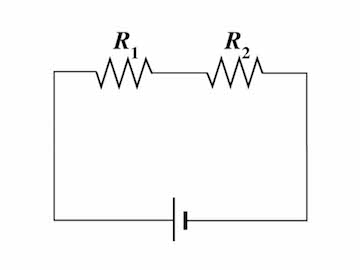# Resistors!In the figure above, two resistors $R_1= 36.0\ \Omega$ and $R_2=18.0\ \Omega$ are connected in series across a $15.0\text{ V}$ battery.

What is the total voltage across the two resistors?

×

Problem Loading...

Note Loading...

Set Loading...